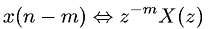Equations > Signal Processing > Z Transform Properties > Z-transform translation (time shift) property

### Z-transform translation (time shift) propertyLatex Code:

MathML Code:

 $x\left(n-m\right)⇔{z}^{-m}X\left(z\right)$

MathType 5.0: